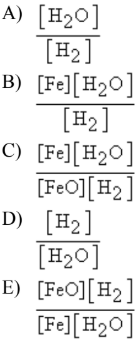# Problem: What is the Kc equilibrium-constant expression for the following equilibrium?FeO(s) + H2(g) ⇌ Fe(s) + H2O(g)

###### FREE Expert Solution

We're being asked to write the expression for the equilibrium constant Kc for the following reaction:

FeO(s) + H2(g) ⇌ Fe(s) + H2O(g)

Since the reaction is balanced, we can proceed to write the Kp expression. Recall that the equilibrium constant is the ratio of the products and reactants

We use Kp when dealing with pressure and Kc when dealing with concentration:

$\overline{){{\mathbf{K}}}_{{\mathbf{p}}}{\mathbf{=}}\frac{{\mathbf{P}}_{\mathbf{products}}}{{\mathbf{P}}_{\mathbf{reactants}}}}$     $\overline{){{\mathbf{K}}}_{{\mathbf{c}}}{\mathbf{=}}\frac{\mathbf{\left[}\mathbf{products}\mathbf{\right]}}{\mathbf{\left[}\mathbf{reactants}\mathbf{\right]}}}$

99% (494 ratings)###### Problem Details

What is the Kc equilibrium-constant expression for the following equilibrium?

FeO(s) + H2(g) ⇌ Fe(s) + H2O(g)